## Galilean:x' with respect to S'? AND SPECIAL BONUS x' = x-d?

Things that don't belong anywhere else. (Check first).

Moderators: Moderators General, Prelates, Magistrates

eSOANEM
:D
Posts: 3652
Joined: Sun Apr 12, 2009 9:39 pm UTC
Location: Grantabrycge

### Re: We're on the road to nowhere

steve waterman wrote:Given only Cartesian system S(x,y,z), x IS mathematically defined, imo.

And your opinion is incorrect in that. Mathematically, it is incredibly important that the objects we consider are always separate from the labels we assign them. As such, we can rename our variables whatever we like. We can call "x" "u" if we want. We can call "x" "1-a^2" we can call it "the abscissa" we can call it whatever we like provided we don't use the same name for two different objects.

This is true not just of variables, but of points. We can call a point P, we can also call it Q or A or Z or whatever. We can also call it by some systematic name such as (1,0,3) or (5,2,1) and all of these can refer to the same point. A co-ordinate system is just something that tells you the name of an arbitrary point without having to name each individually. We have to define what these points are though (and therefore specifying the co-ordinate system when we give co-ordinates as the name of the point).
my pronouns are they

Magnanimous wrote:(fuck the macrons)

steve waterman
Posts: 1610
Joined: Mon Nov 14, 2011 4:39 pm UTC

### Re: Galilean:x' with respect to S'? AND SPECIAL BONUS x' = x

http://mathworld.wolfram.com/Abscissa.html
The x- (horizontal) coordinate of a point in a two dimensional coordinate system. Physicists and astronomers sometimes use the term to refer to the axis itself instead of the distance along it.

That is big part of the problem , your x sometimes means the x axis to you and/or sometimes a distance/number!

Are you allowing me to place S' axis as the label, as shown in the previously posted depiction?
"While statistics and measurements can be misleading, mathematics itself, is not subjective."
"Be careful of what you believe, you are likely to make it the truth."
steve

Vetala
Posts: 91
Joined: Sat Jul 28, 2012 9:07 pm UTC

### Re: Galilean:x' with respect to S'? AND SPECIAL BONUS x' = x

steve waterman wrote:http://mathworld.wolfram.com/Abscissa.html
The x- (horizontal) coordinate of a point in a two dimensional coordinate system. Physicists and astronomers sometimes use the term to refer to the axis itself instead of the distance along it.

That is big part of the problem , your x sometimes means the x axis to you and/or sometimes a distance/number!

Something having different meanings isn't a problem at all. That's why context is important. If I say "salmon" without context I might mean a fish, I might mean part of that fish on my grill, I might mean part of that fish in a can, or a might mean a color. And yet, somehow, the word "salmon" doesn't cause problems in day to day use. That somehow is because there's context attached.

And *that* is (one reason anyway) why nobody wants to let you away with swinging about x'=x-vt while removing all context, because IN THE INTENDED CONTEXT it makes perfect sense - and I refuse to believe that you can't see that, you just can't admit it (for some reason). Taken out of its intended context and every term and surrounding concept either stripped or misused... well... apparently my salmon walls are made out of fish meat?yurell
Posts: 2924
Joined: Sat Nov 13, 2010 2:19 am UTC
Location: Australia!

### Re: Galilean:x' with respect to S'? AND SPECIAL BONUS x' = x

Steve, we've said 'x-axis' every time we've meant 'x-axis'. We've been rather strict in our notation, so that we can communicate more readily with you.
cemper93 wrote:Dude, I just presented an elaborate multiple fraction in Comic Sans. Who are you to question me?

Pronouns: Feminine pronouns please!

Schrollini
Posts: 515
Joined: Sat Sep 29, 2012 5:20 pm UTC

### Ride a painted pony

steve waterman wrote:http://mathworld.wolfram.com/Abscissa.html
The x- (horizontal) coordinate of a point in a two dimensional coordinate system. Physicists and astronomers sometimes use the term to refer to the axis itself instead of the distance along it.

That is big part of the problem , your x sometimes means the x axis to you and/or sometimes a distance/number!

It sounds like it would have been nice if someone had written a long post describing our sloppiness in sometimes using x as part of the name of a coordinate system and sometimes using it to refer to a specific point's coordinate. That someone could have taken the time to point out that this gives rise to two very different ways of writing coordinate transformations and given some examples to show how to translate from one language to another.

Man, if only that had happened!
For your convenience: a LaTeX to BBCode converter

Dark Avorian
Posts: 546
Joined: Mon May 03, 2010 10:48 pm UTC

### Re: Galilean:x' with respect to S'? AND SPECIAL BONUS x' = x

I don't know why I'm doing this. But:

I will herein attempt to explain, in a single post, what x' = x -vt means to me.

This equation occurs in the context of a four dimensional environment which we may just as well think of purely mathematically. This environment (really this manifold, but you apparently can't deal with that), contains points (known as events), which require four coordinates to be identified.

We consider two separate coordinate systems on this environment: namely S and S', each of which identify 4-tuples of coordinates with points in the environment, in a unique way.

If we let P be an event, it has coordinates (x,y,z,t) under system S. It has coordinates (x',y',z',t') under S'.

That is to say: P = S(x,y,z,t) = S'(x',y',z',t')

Now, we know, for simplicities sake, the following things:

(i) For any point P = S(x,y,z,t) = S'(x',y',z',t'), we assume that: t=t' (this is peculiar to the Galilean, and what relativity says is wrong)

(ii) For any point P = S(x,y,z,t) = S'(x',y',z',t'), we assume that: y' = y, z' = z (this is an easy simplification, we can just rotate axes so that the change between systems is in one direction (supposing their relation is simple)

(iii) IF a point P is SUCH THAT t = t' = 0, then: x = x' (this is the coincidence, but note that it only applies for t=0

In this context, we relate the two coordinate representations of P as follows: We know immediately from (i), (ii) that y=y', z=z', t=t'. We further relate the coordinates of P as follows: We may write x', which is one coordinate of P in S, that x' = x - vt for some fixed v, and all P, given that we know the time coordinate of P as well.

NOTE: identifying a point P requires identifying its time coordinate. We have no way of identifying stationary points, however we can certainly identify events. Consider the skew axes I posted at one point, try and draw a line that has constant abscissa in both coordinate systems (hint, you cannot). This is what you are trying to do by equating coordinate systems after changing the t coordinate.

To all others reading the thread, if you agree with this explanation, and would like to try and get steve to actually respond to this post, say so in your posts.
The 62-foot tall statue of Jesus constructed out of styrofoam, wood and fiberglass resin caught on fire after the right hand of the statue was struck by lightning.

meatyochre wrote:And yea, verily the forums crowd spake: "Teehee!"

beojan
Posts: 166
Joined: Mon May 23, 2011 12:11 pm UTC
Location: Oxford / London, United Kingdom, Europe

### Re: Galilean:x' with respect to S'? AND SPECIAL BONUS x' = x

steve waterman wrote:http://mathworld.wolfram.com/Abscissa.html
The x- (horizontal) coordinate of a point in a two dimensional coordinate system. Physicists and astronomers sometimes use the term to refer to the axis itself instead of the distance along it.

That is big part of the problem , your x sometimes means the x axis to you and/or sometimes a distance/number!

Are you allowing me to place S' axis as the label, as shown in the previously posted depiction?

If you look again, that is the definition of "abscissa", not the definition of "x".
That is, it is "abscissa" that can sometimes refer to the axis, or to the horizontal coordinate. If you look back, I have stated before that using the "abscissa, ordinate, applicate" terminology may be part of why you are confused. It is you who keeps using the term abscissa, not the rest of us.

JudeMorrigan
Posts: 1266
Joined: Tue Jan 26, 2010 1:26 pm UTC

### Re: Galilean:x' with respect to S'? AND SPECIAL BONUS x' = x

beojan wrote:1. You either do not have, or have forgotten, mathematics at basic algebra level (what in the UK would be year 8 or 9 / middle school in the US) and above
or 2. You have realized at some point that your ideas regarding special relativity, etc are wrong, but continue to argue, in bad faith, in the hope of avoiding embarassment.

Oh, he's arguing in bad faith, but it's not to try to avoid embarrassment. He admitted in the first thread that he has a pet theory of everything that has to do with closely-packed spheres, that someone managed to convince him that relativity was evidence against his theory, and that he went deliberately to try to find some flaw in relativity to save his theory. Quoth steve: "I had a book with in the transformations with lorentz too, so I started with x' = x-vt, as it does...and worked out ifthe math was okay in each equation...it was. So i concluded that the only chance of it being wrong would have to be that equation."

steve waterman
Posts: 1610
Joined: Mon Nov 14, 2011 4:39 pm UTC

### Re: Galilean:x' with respect to S'? AND SPECIAL BONUS x' = x

AGAIN. NO time, no physics, just case 1 and case 2...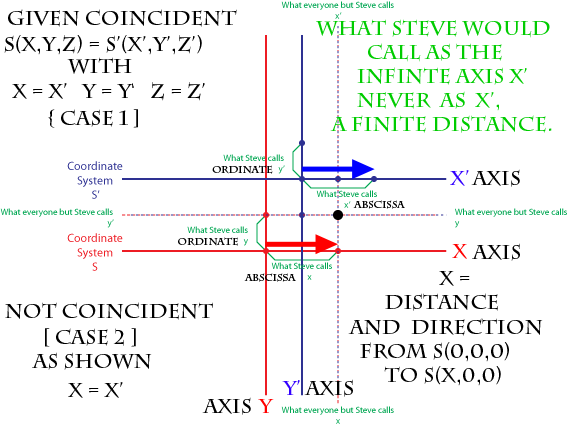CASE 1 spatially coincident*
x = the x axis? or abscissa x?

CASE 2 spatially non-coincident*
your initial depiction displays a common x/x' axis...
so x axis = x' axis in case 2?

*http://www.thefreedictionary.com/coincident
Occupying the same area in space or happening at the same time:

In MY challenge, there is no time element. In the Galilean there is a time element.
I am not discussing anything Galilean, any more in this thread, as I have mentioned.

This is ALL coming down to ...given x = x', means?
Obviously, then given x = x' means the infinite axis x = the infinite axis x'...for all of you.

Obviously too, if x = x' mathematically meant that the x abscissa = x' abscissa, then you WOULD accept that a mathematical inequality, was being generated, should we not end up with x abscissa = x' abscissa in a transformation process.

question - does the larger black dot represent the one origin (0,0,0) of your manifold,
since your x axis = your x' axis and intersects where your y axis = y' axis?

OBSERVATION: case 2 always has x = x', x either taken to mean abscissa or x taken to mean x axis.

That is all I am going for. Given x = x', after any transformation x = x'.
"While statistics and measurements can be misleading, mathematics itself, is not subjective."
"Be careful of what you believe, you are likely to make it the truth."
steve

Dark Avorian
Posts: 546
Joined: Mon May 03, 2010 10:48 pm UTC

### Re: Galilean:x' with respect to S'? AND SPECIAL BONUS x' = x

x' =x -vt refers to the space and time coordinates of A SINGLE EVENT. NOT A POINT IN SPACE OVER TIME.

Also, steve. Suppose I said that when y = 0, then x = x'. Does that always mean that the x and x' axis are in the same place.?
The 62-foot tall statue of Jesus constructed out of styrofoam, wood and fiberglass resin caught on fire after the right hand of the statue was struck by lightning.

meatyochre wrote:And yea, verily the forums crowd spake: "Teehee!"

steve waterman
Posts: 1610
Joined: Mon Nov 14, 2011 4:39 pm UTC

### Re: Galilean:x' with respect to S'? AND SPECIAL BONUS x' = x

Dark Avorian wrote:x' =x -vt refers to the space and time coordinates of A SINGLE EVENT. NOT A POINT IN SPACE OVER TIME.

Also, steve. Suppose I said that when y = 0, then x = x'. Does that always mean that the x and x' axis are in the same place.?

I do not seem to be making this clear. MY CHALLENGE...
NO EVENTS. NO TIME COORDINATE. NO POINTS.
I am not challenging x' = x-vt. I am saying that given x = x', therefore x = x'.

Dark Avorian wrote:when y = 0

then there is no x or x' nor S nor S' nor a coordinate system...
you only have y = 0. y is not a coordinate, all by itself.

GIVEN S(x,y,z) spatially coincident with S'(x',y',z') and x = x', y = y', z = z',
where x can mean either the abscissa x or the x axis,
it remains true that x = x', even if the two systems are no longer spatially coincident.
"While statistics and measurements can be misleading, mathematics itself, is not subjective."
"Be careful of what you believe, you are likely to make it the truth."
steve

firechicago
Posts: 621
Joined: Mon Jan 11, 2010 12:27 pm UTC
Location: One time, I put a snowglobe in the microwave and pushed "Hot Dog"

### Re: Galilean:x' with respect to S'? AND SPECIAL BONUS x' = x

steve waterman wrote:
it remains true that x = x', even if the two systems are no longer spatially coincident.

If x and x' are the coordinates in two systems of the same point, then this statement is completely, entirely, manifestly false, and repeating it will not make it true. If the coordinate system S' changes (for example by moving its origin so that it is no longer coincident with S) then the coordinate x' for a given point will also change, so that x no longer equals x'.
Last edited by firechicago on Sat Jul 27, 2013 2:37 pm UTC, edited 1 time in total.

ucim
Posts: 6888
Joined: Fri Sep 28, 2012 3:23 pm UTC
Location: The One True Thread

### Re: Galilean:x' with respect to S'? AND SPECIAL BONUS x' = x

steve waterman wrote:This is ALL coming down to ...given x = x', means?
Obviously, then given x = x' means the infinite axis x = the infinite axis x'...for all of you.
x and x' are variables. They stand for real numbers.

x=x' means that for every value of x you can choose, the value of x' will be the same. Context may limit this, for example if the expression is used in an if statement.

The phrase:
given x=x'
to me means the same thing as:
if x=x'

That is to say, everything that follows depends on this equality. If something you say breaks that equality, it cannot be true.

As a consequence, if you start with:
given x=x'
and then say
"Now we move the coordinate system..."
you break that equality.

You cannot "move" the coordinate system under those circumstances. You are instead considering a different system in which x does not equal x'

steve waterman wrote:question - does the larger black dot represent the one origin (0,0,0) of your manifold,
No. A thousand times no.

The manifold does not have an origin. Only the coordinate system does. Different coordinate systems can have different manifolds.

The large black dot represents Peter, the point about which we are talking. The point that has different coordinates in different systems. The point whose first coordinate is x in one system, x' in the other system.

After you have "moved" the coordinate system, x no longer equals x' even though in both cases they are the first coordinate of the same point.

Read the first part of this post to see why.

Jose
Order of the Sillies, Honoris Causam - bestowed by charlie_grumbles on NP 859 * OTTscar winner: Wordsmith - bestowed by yappobiscuts and the OTT on NP 1832 * Ecclesiastical Calendar of the Order of the Holy Contradiction * Heartfelt thanks from addams and from me - you really made a difference.

steve waterman
Posts: 1610
Joined: Mon Nov 14, 2011 4:39 pm UTC

### Re: Galilean:x' with respect to S'? AND SPECIAL BONUS x' = x

firechicago wrote:
steve waterman wrote:GIVEN S(x,y,z) spatially coincident with S'(x',y',z') and x = x', y = y', z = z',
where x can mean either the abscissa x or the x axis,
it remains true that x = x', even if the two systems are no longer spatially coincident.

If x and x' are the coordinates in two systems of the same point, then this statement is completely, entirely, manifestly false, and repeating it will not make it true. If the coordinate system S' changes (for example by moving its origin so that it is no longer coincident with S) then the coordinate x' for a given point will also change, so that x no longer equals x'.

GIVEN S(x,y,z) spatially coincident with S'(x',y',z') and x = x', y = y', z = z',
There are NO points.

There is case 1 and case 2.
"While statistics and measurements can be misleading, mathematics itself, is not subjective."
"Be careful of what you believe, you are likely to make it the truth."
steve

JudeMorrigan
Posts: 1266
Joined: Tue Jan 26, 2010 1:26 pm UTC

### Re: Galilean:x' with respect to S'? AND SPECIAL BONUS x' = x

steve waterman wrote:I do not seem to be making this clear. MY CHALLENGE...
NO EVENTS. NO TIME COORDINATE. NO POINTS.
I am not challenging x' = x-vt. I am saying that given x = x', therefore x = x'.

And we do not seem to be making this clear - your "challenge" is meaningless gibberish. There's a reason I keep coming comparing it to Jabberwocky. It's not that we're dense. It's not that we're unwilling to give you a fair shot. It's that your arguments are literal non-sense.

steve waterman
Posts: 1610
Joined: Mon Nov 14, 2011 4:39 pm UTC

### Re: Galilean:x' with respect to S'? AND SPECIAL BONUS x' = x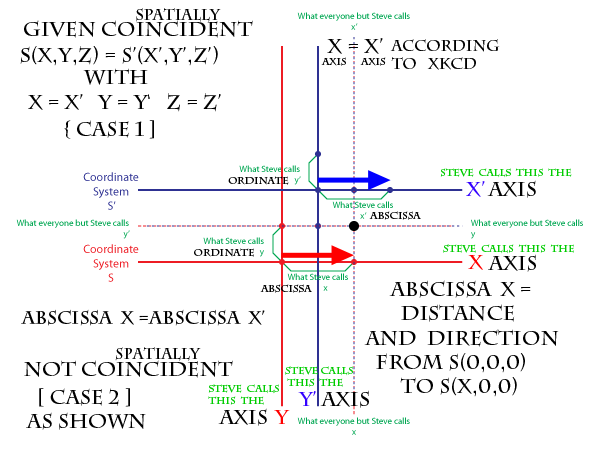ADDED-

GIVEN S(x,y,z) spatially coincident with S'(x',y',z') and x = x', y = y', z = z', ( CASE 1)
where x can mean either the abscissa x or the x axis,
it remains true that x = x', even if the two systems are no longer spatially coincident ( CASE 2).

added -
so, the black dot is point P in the manifold. Point P is located at the juncture of your
x axis = x' axis with the
y axis = y' axis with the
z axis = z' axis
and yet point P is not located at (0,0,0) in the manifold because your manifold has no origin.
The manifold has a point P, which is mapped from both S(x,y,z) and S'(x',y',z')....correct?
"While statistics and measurements can be misleading, mathematics itself, is not subjective."
"Be careful of what you believe, you are likely to make it the truth."
steve

JudeMorrigan
Posts: 1266
Joined: Tue Jan 26, 2010 1:26 pm UTC

### Re: Galilean:x' with respect to S'? AND SPECIAL BONUS x' = x

The dashed lines aren't axes, Steve. You're misunderstanding what they're supposed to represent.

ucim
Posts: 6888
Joined: Fri Sep 28, 2012 3:23 pm UTC
Location: The One True Thread

### Re: Galilean:x' with respect to S'? AND SPECIAL BONUS x' = x

steve waterman wrote:it remains true that x = x', even if the two systems are no longer spatially coincident ( CASE 2).
No.
That's the whole point.

Also, the purpley dashed lines are not axes. They are simply visual aids to help people locate the value of the coordinates in different systems. Follow a purpley dashed line to an axis, and read off the value at that axis, in that system. If you follow it to one system's axis, you'll get the value of x (or y, depending on which dimension you're looking at). If you follow it to the other system's axis, you'll get the value of x' (or y'). In each case, the value you get will be the respective coordinate of the point, in that system.

You'll notice that those values (x and x') are different. Yet they relate to the same point.

steve waterman wrote:The manifold has a point P, which is mapped from both S(x,y,z) and S'(x',y',z')....correct?
Yes

Jose
Order of the Sillies, Honoris Causam - bestowed by charlie_grumbles on NP 859 * OTTscar winner: Wordsmith - bestowed by yappobiscuts and the OTT on NP 1832 * Ecclesiastical Calendar of the Order of the Holy Contradiction * Heartfelt thanks from addams and from me - you really made a difference.

eSOANEM
:D
Posts: 3652
Joined: Sun Apr 12, 2009 9:39 pm UTC
Location: Grantabrycge

### Re: Galilean:x' with respect to S'? AND SPECIAL BONUS x' = x

steve waterman wrote:
Dark Avorian wrote:x' =x -vt refers to the space and time coordinates of A SINGLE EVENT. NOT A POINT IN SPACE OVER TIME.

Also, steve. Suppose I said that when y = 0, then x = x'. Does that always mean that the x and x' axis are in the same place.?

I do not seem to be making this clear. MY CHALLENGE...
NO EVENTS. NO TIME COORDINATE. NO POINTS.
I am not challenging x' = x-vt. I am saying that given x = x', therefore x = x'.

That statement's trivial.

I don't know why you think anyone is or would argue against it.
my pronouns are they

Magnanimous wrote:(fuck the macrons)

firechicago
Posts: 621
Joined: Mon Jan 11, 2010 12:27 pm UTC
Location: One time, I put a snowglobe in the microwave and pushed "Hot Dog"

### Re: Galilean:x' with respect to S'? AND SPECIAL BONUS x' = x

steve waterman wrote:GIVEN S(x,y,z) spatially coincident with S'(x',y',z') and x = x', y = y', z = z',
There are NO points.

There is case 1 and case 2.

If there are no points then S and S' are not coordinate systems (as I mentioned earlier, a coordinate system is a mapping of coordinates onto a set of points) and talking about them being coincident or moving is nothing but gibberish.

steve waterman
Posts: 1610
Joined: Mon Nov 14, 2011 4:39 pm UTC

### Re: Galilean:x' with respect to S'? AND SPECIAL BONUS x' = x

ucim wrote:
steve waterman wrote:it remains true that x = x', even if the two systems are no longer spatially coincident ( CASE 2).
No.
That's the whole point.

Also, the purpley dashed lines are not axes. They are simply visual aids to help people locate the value of the coordinates in different systems. Follow a purpley dashed line to an axis, and read off the value at that axis, in that system. If you follow it to one system's axis, you'll get the value of x (or y, depending on which dimension you're looking at). If you follow it to the other system's axis, you'll get the value of x' (or y'). In each case, the value you get will be the respective coordinate of the point, in that system.

You'll notice that those values (x and x') are different. Yet they relate to the same point.

steve waterman wrote:The manifold has a point P, which is mapped from both S(x,y,z) and S'(x',y',z')....correct?
Yes

Jose

the dashed lines, what you all call x....is not an axis?
ucim wrote:so x is read off the value [b]at that axis, in that system,

an infinite vertical reference line that maps distances from the yz plane, but is not defined as an axis.

Please then, can you define what your x is then,
as the first coordinate of the S(x,y,z) mapped to point P in the manifold?
I really would love a concrete mathematical definition of what YOUR x means, and I would really like to stop guessing at what it might possibly be or not be; point,abscissa,axis,number,distance,vector,etc, etc
having x labeled as part of the depiction is certainly helpful to try and understand what you all mean by x.

x as xkcd has labeled, is the dotted infinite vertical reference line.
The dotted infinite vertical reference line is mapped to, but not inherent to, your manifold. Correct?

I do grasp that P has different coordinates in the manifold, as I did shortly after the manifold issue first came up.
Getting YOUR notation posted for that formula, I found to be amazingly difficult to get as feedback.
I am feeling that getting a clear definition of what your x means seems to be following that trail.

Not one comment, so far, about my labeling the infinite horizontal x axis
as opposed to the xkcd infinite vertical reference line as x, (but not an axis.)
"While statistics and measurements can be misleading, mathematics itself, is not subjective."
"Be careful of what you believe, you are likely to make it the truth."
steve

ucim
Posts: 6888
Joined: Fri Sep 28, 2012 3:23 pm UTC
Location: The One True Thread

### Re: Galilean:x' with respect to S'? AND SPECIAL BONUS x' = x

steve waterman wrote:the dashed lines, what you all call x....is not an axis?
You are misreading the "what everyone but Steve calls..." part.

If you look at one side, it says " what everyone but Steve calls x ". (just plain old x)
If you look at the other side, it says " what everyone but Steve calls x' ". (x prime)

What it refers to is not the purpley line itself. Rather, take a look at where that purpley line intersects the respective axis of the red or the blue system. That is what is being referred to. It's a different point (on that axis) from the one indicated by "What Steve calls...".

steve waterman wrote:Please then, can you define what your x is then,
x is a variable. It is a placeholder for a value. In the context of this diagram, it is the placeholder for the value of the first coordinate of a point P, when expressed in the red system.

Similarly,
x' is a variable. It is a placeholder for a value. In the context of this diagram, it is the placeholder for the value of the first coordinate of a point P, when expressed in the blue system.

"The x axis" is the locus of points in the (in this case 2D) manifold whose the value of the second coordinate (typically called y) is zero, when expressed in the red system.

steve waterman wrote:Not one comment, so far, about my labeling the infinite horizontal x axis
as opposed to the xkcd infinite vertical reference line as x, (but not an axis.)
Notice the definition of x axis above. In an x-y plane,
the x axis is a line of constant y (where the constant it equals is zero).
the y axis is a line of constant x (where the constant it equals is zero).

Now, a line of constant x, where the constant is not zero, is also a vertical line (in standard notation). Thus, it is also parallel to the y axis.

So, the line "x=3" is a vertical line, parallel to the y axis, offset from it by three units.

Jose
Order of the Sillies, Honoris Causam - bestowed by charlie_grumbles on NP 859 * OTTscar winner: Wordsmith - bestowed by yappobiscuts and the OTT on NP 1832 * Ecclesiastical Calendar of the Order of the Holy Contradiction * Heartfelt thanks from addams and from me - you really made a difference.

Pfhorrest
Posts: 5474
Joined: Fri Oct 30, 2009 6:11 am UTC
Contact:

### Re: Galilean:x' with respect to S'? AND SPECIAL BONUS x' = x

Just a comment for clarity: there are actually two dashed blue lines, and two dashed red lines, but because they lie in the same place on the diagram they look purpley from a distance. Maybe this will be clearer: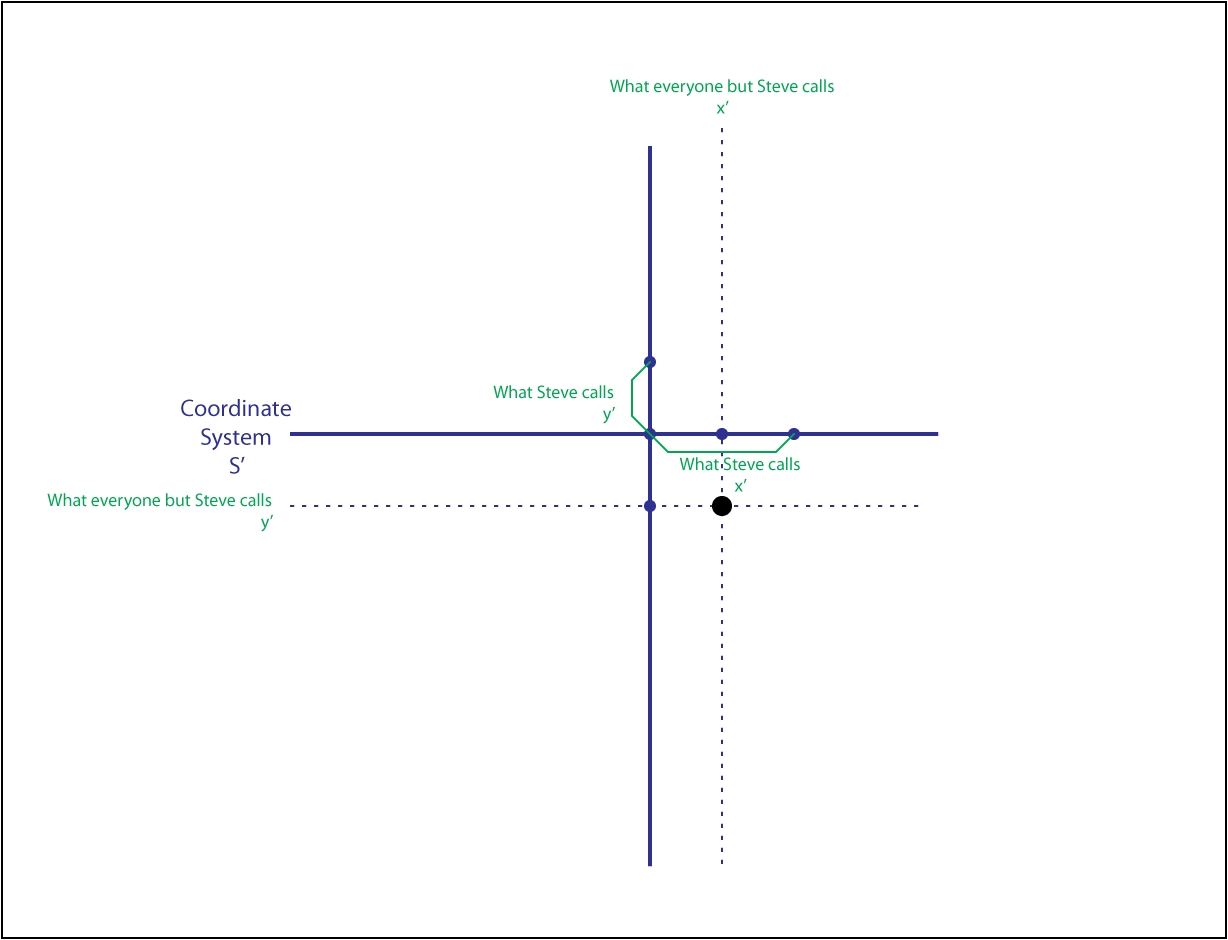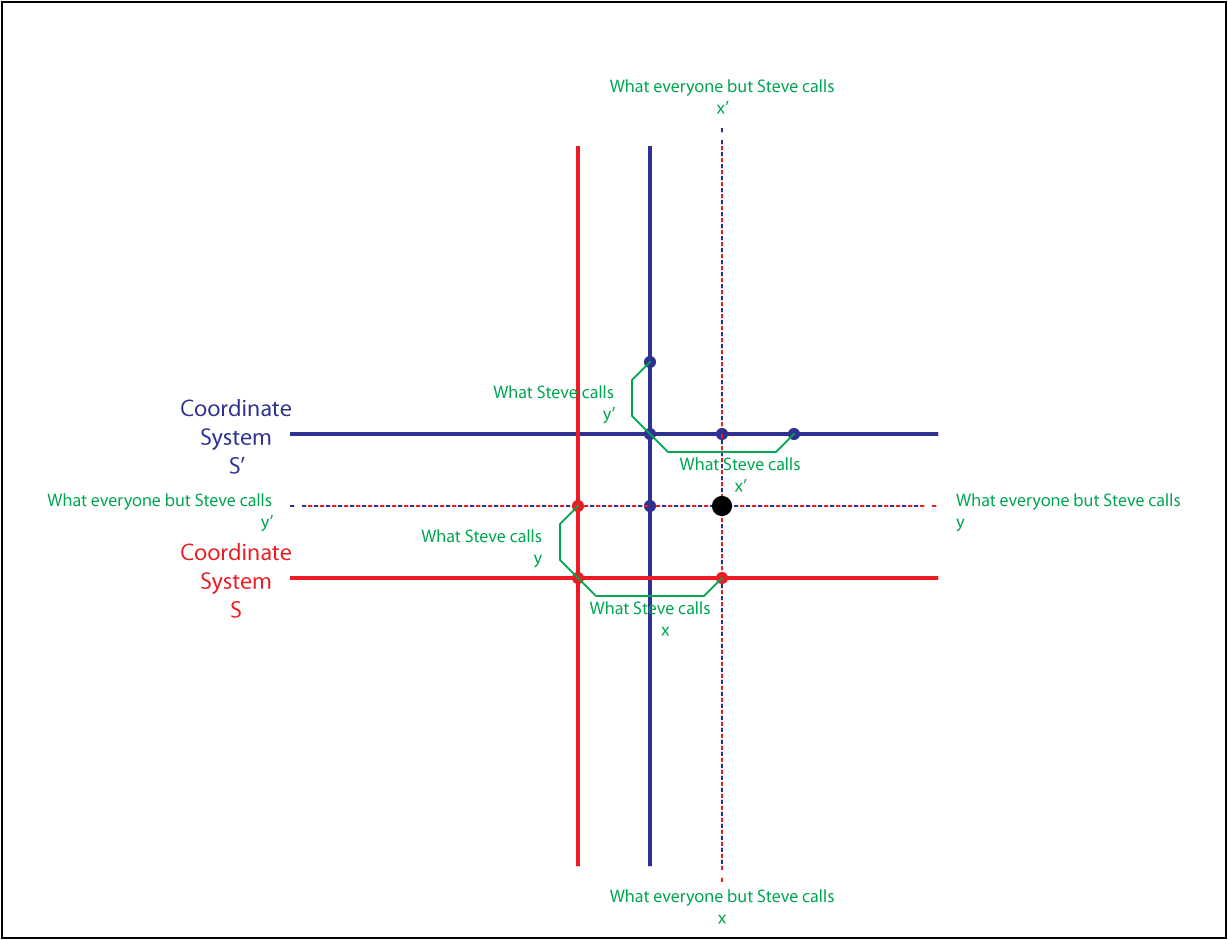In particular, Steve, look at that second diagram there. Note how your so-called x' and y', being distances along the horizontal and vertical axes of the S' system, have no connection whatsoever to the point under consideration (the black dot), because of the roundabout way you define them via x and y and their relationship via the S system to that point under consideration.

[EDIT: "dashed" not "dashes"][EDIT 2: Finish sentence I cut short when posting in a hurry earlier]
Last edited by Pfhorrest on Sun Jul 28, 2013 1:58 am UTC, edited 2 times in total.
Forrest Cameranesi, Geek of All Trades
"I am Sam. Sam I am. I do not like trolls, flames, or spam."
The Codex Quaerendae (my philosophy) - The Chronicles of Quelouva (my fiction)

steve waterman
Posts: 1610
Joined: Mon Nov 14, 2011 4:39 pm UTC

### Re: Galilean:x' with respect to S'? AND SPECIAL BONUS x' = x

Just checking to see if these group of statements below are correct...

In a Cartesian coordinate system, given S(x,y,z), the x axis, y axis, and z axis intersect at S(0,0,0).

given S(x,y,z)....Physics takes x, by itself, to mean the x axis.

given S(x,y,z)....Mathematics traditionally takes x, by itself, to mean a finite value.

given S(x,y,z)...The x axis is inherent to S.
given S(x,y,z)...the x axis is not inherent to the manifold. In the manifold, the x axis is mapped from the inherent S.

given S(x,y,z) coincident with S'(x',y',z'),
there is no x axis inherent to the S' system,
( nor by virtue of a transformation nor mapping nor as any given nor otherwise. )

added -
Schrollini wrote:no connection whatsoever to the point under consideration (the black dot).

CORRECT.
xkcd equates coordinate TRIPLETS for point P in the manifold. Fine, I totally agree. I have agreed for months.

if we concentrate/focus/isolate upon just the red S system and just the blue S' system...
x abscissa = x' abscissa in both case 1 and case 2.
if we concentrate/focus/isolate upon point P in the manifold,
the coordinates of point P are the same for S and S' in case 1.
the coordinates of point P differ when mapped from S and S' in case 2.

edit - to the last two lines

added - AND NOW EDIT/REPLACE THE DEPICTION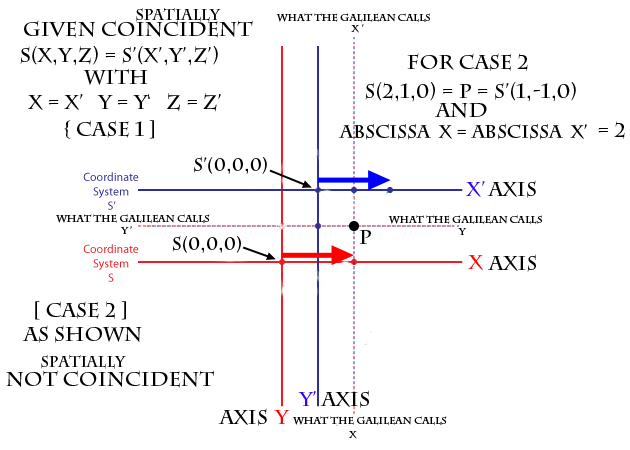"While statistics and measurements can be misleading, mathematics itself, is not subjective."
"Be careful of what you believe, you are likely to make it the truth."
steve

Pfhorrest
Posts: 5474
Joined: Fri Oct 30, 2009 6:11 am UTC
Contact:

### Re: Galilean:x' with respect to S'? AND SPECIAL BONUS x' = x

Animated version of those last three diagrams for even greater clarity:Forrest Cameranesi, Geek of All Trades
"I am Sam. Sam I am. I do not like trolls, flames, or spam."
The Codex Quaerendae (my philosophy) - The Chronicles of Quelouva (my fiction)

yurell
Posts: 2924
Joined: Sat Nov 13, 2010 2:19 am UTC
Location: Australia!

### Re: Galilean:x' with respect to S'? AND SPECIAL BONUS x' = x

Steve, if x=x', then x=x'. Given this, what is your point?! Nobody will disagree with this trivially true statement.
However, if you make it for all co-ordinates, then you've only performed a trivial transformation — your two co-ordinate systems are the same. Given this, why do you want to discuss it? Everyone else is contesting the statement x=x' because no one else is talking about two identical co-ordinate systems. You can tell this by the diagrams that everyone shows.

So just to be sure, I have two questions:
1) Are you talking about identical co-ordinate systems?
YES: We are done. Everyone agrees that if the co-ordinate systems are identical, then x=x', y=y', z=z' and t=t'.
NO: Proceed to question 2.
2) Can you explain what the equation would be to relate u and u', if the origin of the S'(u') system is d units to the right of the origin of the S(u) system, and S'(u') = S(u) (that is, both map to the same point P).
If you want me to write the answer, just say so and I'll provide it.
cemper93 wrote:Dude, I just presented an elaborate multiple fraction in Comic Sans. Who are you to question me?

Pronouns: Feminine pronouns please!

ucim
Posts: 6888
Joined: Fri Sep 28, 2012 3:23 pm UTC
Location: The One True Thread

### Re: Galilean:x' with respect to S'? AND SPECIAL BONUS x' = x

steve waterman wrote:Just checking to see if these group of statements below are correct...

Corrected versions below:

In a Cartesian coordinate system, given S(x,y,z) and a suitable manifold, the x axis, y axis, and z axis intersect at S(0,0,0).
--> correct as is. The point S(0,0,0) is called the origin of S.

given S(x,y,z)....Physics takes x, by itself, to mean the x axis.
--> no. Physics uses mathematics, and uses the same definitions as Math does.

given S(x,y,z)....Mathematics traditionally takes x, by itself, to mean a placeholder for a finite value.
--> Depending on context, x can be a single (often unknown) value, or the entire set of values which satisfies a condition. For the purposes of these discussions, think of it as a placeholder.

given S(x,y,z)...The x axis is inherent to S.
--> yes. It's part of the definition of S, which establishes the function you are using to identify points.

given S(x,y,z)...the x axis is not inherent to the manifold. In the manifold, the x axis is mapped from the inherent S.
--> Close enough. Better version of your second sentence: The x axis which is inherent to S maps to a set of points in the manifold.

given S(x,y,z) coincident with S'(x',y',z'),
there is no x axis inherent to the S' system,
( nor by virtue of a transformation nor mapping nor as any given nor otherwise. )
--> If you are defining S'(x',y',z'), then the x' axis is inherent to S', the same way the x axis is inherent to S. It is part of the definition of S'.
--> Every point (in the manifold) identified by coordinates in S can also be identified by (different) coordinates in S'
--> As a consequence, every point on the x' axis (of S') also has a corresponding set of coordinates in the S system.
--> In the case of a simple translation of a cartesian coordinate system, there is a simple relationship between the coordinates in S and the coordinates in S' that refer to the same point.

If you understand and agree with the foregoing, I will tell you what that relationship is in the next post.

A word about dimension: The words "abscissa" and "ordinate" ordinarily refer to the x and y axes, respectively, of a two dimensional cartesian system. Once you go past two dimensions, we stop using those words because we'd need another word for each axis, and it gets silly. So long as we are not using the other dimensions in our discussion, there is no loss of generality in considering two dimensional systems, or even one dimensional systems. The principle is the same, and the equation you are having difficulty with is still the same one.

Jose
Order of the Sillies, Honoris Causam - bestowed by charlie_grumbles on NP 859 * OTTscar winner: Wordsmith - bestowed by yappobiscuts and the OTT on NP 1832 * Ecclesiastical Calendar of the Order of the Holy Contradiction * Heartfelt thanks from addams and from me - you really made a difference.

steve waterman
Posts: 1610
Joined: Mon Nov 14, 2011 4:39 pm UTC

### Re: Galilean:x' with respect to S'? AND SPECIAL BONUS x' = x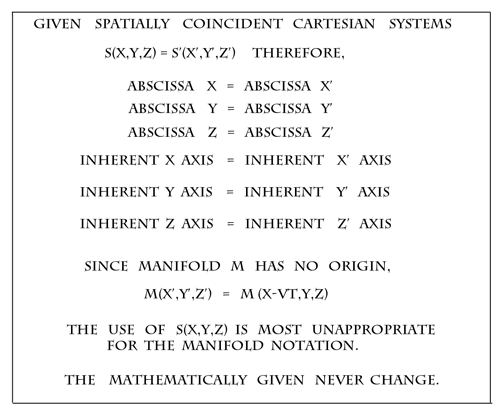added -
ucim - real nice feedback on my list of questions. Thank you so much for the nice clear answers.

Since we are given S(x,y,z), then z generically, ( the third coordinate ) is mathematically called the applicate.
Last edited by steve waterman on Sun Jul 28, 2013 12:16 pm UTC, edited 1 time in total.
"While statistics and measurements can be misleading, mathematics itself, is not subjective."
"Be careful of what you believe, you are likely to make it the truth."
steve

yurell
Posts: 2924
Joined: Sat Nov 13, 2010 2:19 am UTC
Location: Australia!

### Re: Galilean:x' with respect to S'? AND SPECIAL BONUS x' = x

What the hell is M(x,y,z)? Manifolds aren't functions; they get mapped to, they aren't maps themselves.
cemper93 wrote:Dude, I just presented an elaborate multiple fraction in Comic Sans. Who are you to question me?

Pronouns: Feminine pronouns please!

steve waterman
Posts: 1610
Joined: Mon Nov 14, 2011 4:39 pm UTC

### Re: Galilean:x' with respect to S'? AND SPECIAL BONUS x' = x

yurell wrote:What the hell is M(x,y,z)? Manifolds aren't functions; they get mapped to, they aren't maps themselves.

M(x,y,z) are the inherent coordinates from S(x,y,z) mapped to P in the manifold.
M'(x',y',z') are the inherent coordinates from S'(x',y',z') mapped to P in the manifold.

added -
Thanks to all that have resisted posting with issues like time and motion. This is quite appreciated as I know this must be rather annoying.
I think, at last, I have finally presented my issue in a reasonable enough format that others can actually judge the mathematical logic being presented. I needed to have all this feedback from day one, for this recent flurry to be achieved. Noting, it is still to be decided if this challenge is mathematically valid or not. So, now, you get to pick apart everything presented in the proof, piece by piece, if you wish. Please stay with my conditions of no v,t,d, etc.

added again -
I am not directly challenging the Galilean transformations, I am only saying that mathematically given x = x', therefore x = x'.

and added again -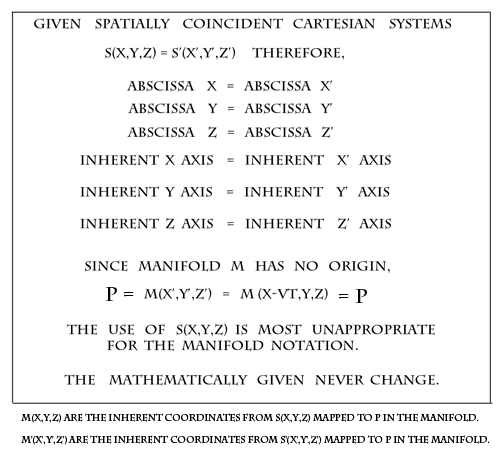added - from page 70 on the pressures thread...
http://forums.xkcd.com/viewtopic.php?f=2&t=96231&p=3132741&hilit=northeastern#p3132741
Some background regarding my math history.
Last edited by steve waterman on Sun Jul 28, 2013 4:54 pm UTC, edited 2 times in total.
"While statistics and measurements can be misleading, mathematics itself, is not subjective."
"Be careful of what you believe, you are likely to make it the truth."
steve

beojan
Posts: 166
Joined: Mon May 23, 2011 12:11 pm UTC
Location: Oxford / London, United Kingdom, Europe

### Re: Galilean:x' with respect to S'? AND SPECIAL BONUS x' = x

steve waterman wrote:
yurell wrote:What the hell is M(x,y,z)? Manifolds aren't functions; they get mapped to, they aren't maps themselves.

M(x,y,z) are the inherent coordinates from S(x,y,z) mapped to P in the manifold.
M'(x',y',z') are the inherent coordinates from S'(x',y',z') mapped to P in the manifold.

This is meaningless. MEANINGLESS.

Steve, have you ever actually been taught any mathematics, by anyone, ever?
Sometimes it seems you string together words in such a way that they make no sense whatsoever as a sentence.

steve waterman
Posts: 1610
Joined: Mon Nov 14, 2011 4:39 pm UTC

### Re: Galilean:x' with respect to S'? AND SPECIAL BONUS x' = x

beojan wrote:
steve waterman wrote:
yurell wrote:What the hell is M(x,y,z)? Manifolds aren't functions; they get mapped to, they aren't maps themselves.

M(x,y,z) are the inherent coordinates from S(x,y,z) mapped to P in the manifold.
M'(x',y',z') are the inherent coordinates from S'(x',y',z') mapped to P in the manifold.

This is meaningless. MEANINGLESS.

Steve, have you ever actually been taught any mathematics, by anyone, ever?
Sometimes it seems you string together words in such a way that they make no sense whatsoever as a sentence.

I posted some of my math background somewhere on the epic thread.

When S(2,0,0) is mapped to P in the manifold, is your point P = S(2,0,0)?

PLEASE write the general equation for what P = in the manifold!
Last edited by steve waterman on Sun Jul 28, 2013 1:47 pm UTC, edited 1 time in total.
"While statistics and measurements can be misleading, mathematics itself, is not subjective."
"Be careful of what you believe, you are likely to make it the truth."
steve

PM 2Ring
Posts: 3715
Joined: Mon Jan 26, 2009 3:19 pm UTC
Location: Sydney, Australia

### Re: Galilean:x' with respect to S'? AND SPECIAL BONUS x' = x

Lasciate ogne speranza, voi ch'intrate.

beojan
Posts: 166
Joined: Mon May 23, 2011 12:11 pm UTC
Location: Oxford / London, United Kingdom, Europe

### Re: Galilean:x' with respect to S'? AND SPECIAL BONUS x' = x

The manifold has no inherent way of addressing points.
You must have a coordinate system in order to address points in the manifold.
However, there is more than one coordinate system that can be used (infinitely many in fact).
There is no privileged coordinate system. Any coordinate system is as valid as any other.
The coordinates of any one point (lets take D as an example) are different in each coordinate system.

Also, could you possibly point me to where you posted about your mathematical background in a previous thread?

ucim
Posts: 6888
Joined: Fri Sep 28, 2012 3:23 pm UTC
Location: The One True Thread

### Re: Galilean:x' with respect to S'? AND SPECIAL BONUS x' = x

steve waterman wrote:ucim - real nice feedback on my list of questions. Thank you so much for the nice clear answers.
Thsnks. Hope they helped.

steve waterman wrote:Since we are given S(x,y,z), then z generically, ( the third coordinate ) is mathematically called the applicate.
That's not what my google-fu comes up with. In any case, new words for each dimension gets unwieldy, so I'll stick with "first coordinate", etc.

As to this image:
Spoiler:It doesn't make sense. Abscissa y and abscissa z contradict the standard meaning of the word "abscissa" (the first coordinate, which you are calling x or x'), and you seem to be trying to make something special about an "inherent" x axis (and y axis, etc). "inherent" is meaningless in this context.

I think you are trying to say that when the axes are coincident, points on the axes have the same coordinates in both systems).
You'll get no argument there... so long as the axes remain coincident. It's when you "move" them that this no longer holds. You alter the "mathematically given" by moving a coordinate system. That's what it means.

Next, the statement:
in a graphic, steve waterman wrote:Since manifold has no origin
P = M(x',t',z') = M(x-vt, y, z) = P
The use of S(x,y,z) is most unappropriate for the manifold notation

is nonsense. Working backwards:
3: there is no such thing as "manifold notation". If you want to refer to a point (in the manifold, which is the only place they are), you need to somehow give it a name. The easiest way to do so is by using a coordinate system; that is what S and S' are, if you define them correctly. S(2,-26,43) is the name of a point in the (3D) manifold, given a suitable definition of S. Q(4,1,-4) is the name of a (probably different) point in the manifold, given a suitable definition of Q. ...

2: ... But you need to give a suitable definition, which includes axes orientation and origin. None has been given for your M, so M(x',y',z') has no meaning. It therefore does not identify a point in the manifold, and the equation
P = M(...)
is not valid.

1: In any case, none of those statements follow from the idea that the manifold has no origin.

Next, you insist that "The mathematically given never change." However, when you (many places elsewhere in the thread) "move the red system" you are in fact changing the mathematically given. This is the piece that breaks your proof.

Jose
Order of the Sillies, Honoris Causam - bestowed by charlie_grumbles on NP 859 * OTTscar winner: Wordsmith - bestowed by yappobiscuts and the OTT on NP 1832 * Ecclesiastical Calendar of the Order of the Holy Contradiction * Heartfelt thanks from addams and from me - you really made a difference.

steve waterman
Posts: 1610
Joined: Mon Nov 14, 2011 4:39 pm UTC

### Re: Galilean:x' with respect to S'? AND SPECIAL BONUS x' = x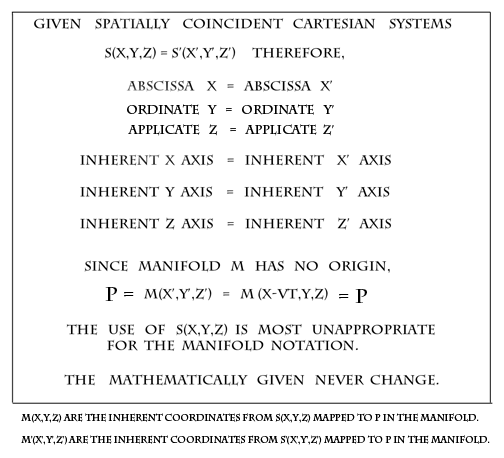Yes, of course, these are all not abscissa, thanks. That was a very silly mistake to make.

edit/added -
Since, M cannot be used to represent the manifold, are we okay with this depiction?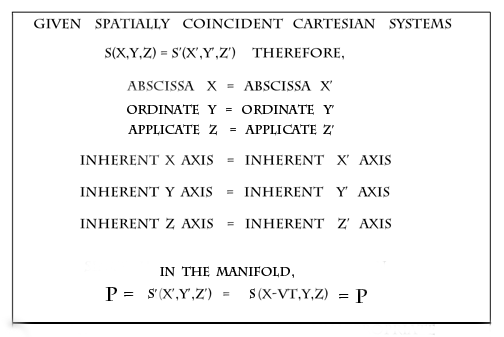ADDED -
Or perhaps, your equation is P = f(x,y,z) = g(x',y',z')?

Pick something please! I really would like to know the formula/equation being used for P in the manifold.
Last edited by steve waterman on Sun Jul 28, 2013 3:36 pm UTC, edited 1 time in total.
"While statistics and measurements can be misleading, mathematics itself, is not subjective."
"Be careful of what you believe, you are likely to make it the truth."
steve

ucim
Posts: 6888
Joined: Fri Sep 28, 2012 3:23 pm UTC
Location: The One True Thread

### Re: Galilean:x' with respect to S'? AND SPECIAL BONUS x' = x

steve waterman wrote:What is the Galilean equation for P in the manifold, please?
Hate to keep you in suspense, but I'm going to first do a different equation, so that we are not referring to v or t. This equation (the one for a simple translation) generalizes easily enough, but I'd like to do it in two stages. I'll also do it in two dimensions (x and y), since we are not going to do anything with z.

Referring back to this post (and ignoring z):

===

Your case #1:
Given S(x,y) and S'(x',y') as cartesian coordinate systems on the same two dimensional manifold M, whose respective axes are coincident with each other,
it can be conlcuded that,
For every point P in the manifold,
--> IF S(x,y) = P
--> AND IF S'(x',y')=P
--> THEN x=x' AND y=y'

This is what "coincident" means.

===

Your case #2, with a specific numerical offset (of 3 horizontal and 7 vertical), as an example:
Given S(x,y) and S"(x",y") (note the double primes, which I'm using to avoid confusion with case #1), as Cartesian coordinate systems on the same two dimensional manifold M, whose respective axes are parallel to each other but are offset from each other by having the origin of the S" system (known as S"(0,0)) being the same point (in the manifold) as the one called S(3,7) in the S system. I'll call this point F. Note that point F (like all points) actually lives in the manifold. The S and S" systems are merely ways to give F different names which are useful in different circumstances.

So,
S"(0,0)= F
S(3,7) = F

Thus, S"(0,0) = S(3,7) = F

THEN, for every point P in the manifold
--> IF S(x,y) = P
--> AND IF S"(x",y") = P
--> THEN x"=x-3 AND y"=y-7

===

Your case #2, with a general numerical offset, represented by parameters c and d:
(Note - I'll still use double-prime notation, but this is a separate case from the one above. New givens.)

Given S(x,y) and S"(x",y") (note the double primes), as Cartesian coordinate systems on the same two dimensional manifold M, whose respective axes are parallel to each other but are offset from each other by having the origin of the S" system (known as S"(0,0)) being the same point (in the manifold) as S(c,d). I'll call this point F. Note that point F (like all points) actually lives in the manifold. The S and S" systems are merely ways to give F different names which are useful in different circumstances.

So,
S"(0,0)= F
S(c,d) = F

Thus, S"(0,0) = S(3,7) = F

THEN, for every point P in the manifold
--> IF S(x,y) = P
--> AND IF S"(x",y") = P
--> THEN x"=x-c AND y"=y-d

If you let c=3 and d=7, then this general case becomes the specific case #2 above.

And, if you let c=0 and d=0, then this general case becomes your case #1 of coincident systems.

Let me know if you follow and agree with this, or where you have a problem with it. The next step will be the actual Galilean, which is similar to this.

Jose
Order of the Sillies, Honoris Causam - bestowed by charlie_grumbles on NP 859 * OTTscar winner: Wordsmith - bestowed by yappobiscuts and the OTT on NP 1832 * Ecclesiastical Calendar of the Order of the Holy Contradiction * Heartfelt thanks from addams and from me - you really made a difference.

steve waterman
Posts: 1610
Joined: Mon Nov 14, 2011 4:39 pm UTC

### Re: Galilean:x' with respect to S'? AND SPECIAL BONUS x' = x

equating x with x',
vt = 3
S(2,0) = P
S'(-1,0) = P
therefore,
2 = -1?

equating point P relative coordinate sets
vt = 3
S(2,0) = P
S'(-1,0) = P
therefore, in the manifold
S(2,0) = S'(-1,0) = P
However, since S(x,y,z) = S'(x',y',z') was given, it is also quite true that S(2,0) = S'(2,0).

So, S(2,0) = S'(2,0) = S'(-1,0)?

edit - took out the quote, which I had misread. The above is stand-alone.

added -
ucim -
The Galilean has no double prime notation/logic, nor do I.
Hence, why I backed down on any possible M(x,y,z)...no such thing.
"While statistics and measurements can be misleading, mathematics itself, is not subjective."
"Be careful of what you believe, you are likely to make it the truth."
steve

ucim
Posts: 6888
Joined: Fri Sep 28, 2012 3:23 pm UTC
Location: The One True Thread

### Re: Galilean:x' with respect to S'? AND SPECIAL BONUS x' = x

steve waterman wrote:equating x with x',
vt = 3
S(2,0) = P
S'(-1,0) = P
therefore,
2 = -1?

equating point P relative coordinate sets
vt = 3
S(2,0) = P
S'(-1,0) = P
therefore, in the manifold
S(2,0) = S'(-1,0) = P
However, since S(x,y,z) = S'(x',y',z') was given, it is also quite true that S(2,0) = S'(2,0).

So, S(2,0) = S'(2,0) = S'(-1,0)?

edit - took out the quote, which I had misread. The above is stand-alone.

Are you attempting to use the same systems that I am?

In your first one, you posit that
S(2,0) = P
S'(-1,0) = P
This could be true of an offset system (such as the one in my S" example) but it is (of course) not true of a coincident system (such as the one in my S' example).

Same problem with the rest of the post. Before you can talk about S and S' and S", they need to be defined. If you are defining them the way I did, then you contradict yourself at the start, because for coincident systems (the ones I used the S and S' for),
S(2,0) = P
S'(-1,0) = P
is not true.

This is why I used double prime notation - to make clear which example we are discussing in each case.

Jose
Order of the Sillies, Honoris Causam - bestowed by charlie_grumbles on NP 859 * OTTscar winner: Wordsmith - bestowed by yappobiscuts and the OTT on NP 1832 * Ecclesiastical Calendar of the Order of the Holy Contradiction * Heartfelt thanks from addams and from me - you really made a difference.

steve waterman
Posts: 1610
Joined: Mon Nov 14, 2011 4:39 pm UTC

### Re: Galilean:x' with respect to S'? AND SPECIAL BONUS x' = x

ucim wrote:
steve waterman wrote:equating x with x',
vt = 3
S(2,0) = P
S'(-1,0) = P
therefore,
2 = -1?

equating point P relative coordinate sets
vt = 3
S(2,0) = P
S'(-1,0) = P
therefore, in the manifold
S(2,0) = S'(-1,0) = P
However, since S(x,y,z) = S'(x',y',z') was given, it is also quite true that S(2,0) = S'(2,0).

So, S(2,0) = S'(2,0) = S'(-1,0)?

edit - took out the quote, which I had misread. The above is stand-alone.

Are you attempting to use the same systems that I am?

In your first one, you posit that
S(2,0) = P
S'(-1,0) = P
This could be true of an offset system (such as the one in my S" example) but it is (of course) not true of a coincident system (such as the one in my S' example).

Same problem with the rest of the post. Before you can talk about S and S' and S", they need to be defined. If you are defining them the way I did, then you contradict yourself at the start, because for coincident systems (the ones I used the S and S' for),
S(2,0) = P
S'(-1,0) = P
is not true.

This is why I used double prime notation - to make clear which example we are discussing in each case.

Jose

ucim wrote:Are you attempting to use the same systems that I am?

NO!CASE1 coincident S and S'
S(2,0) = S'(2,0)

CASE 2 S and S' separated by vt = 3
S(2,0) = S'(-1,0) = P
and
S'(2,0) = S(5,0) = P
"While statistics and measurements can be misleading, mathematics itself, is not subjective."
"Be careful of what you believe, you are likely to make it the truth."
steve

Return to “General”

### Who is online

Users browsing this forum: No registered users and 19 guests